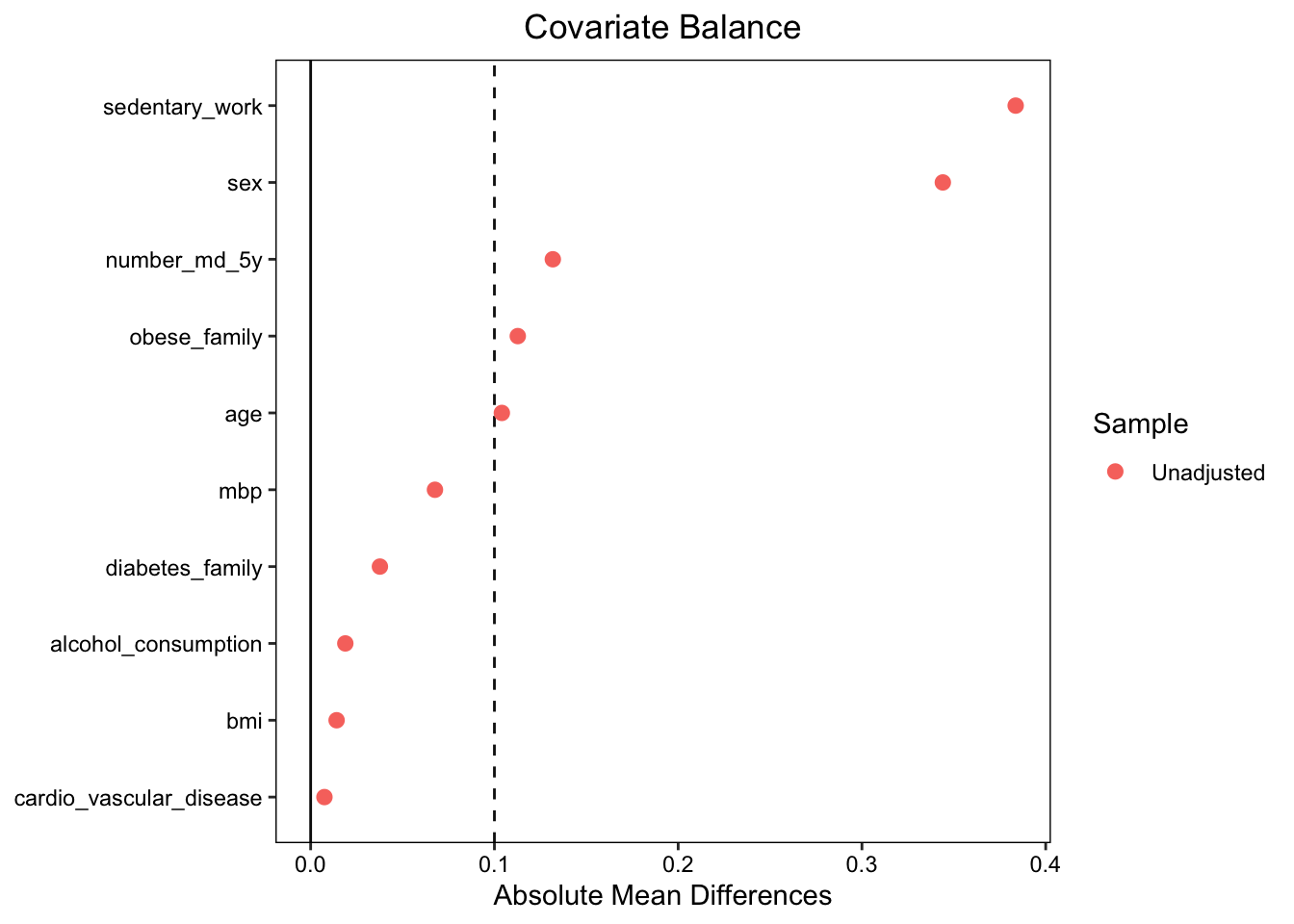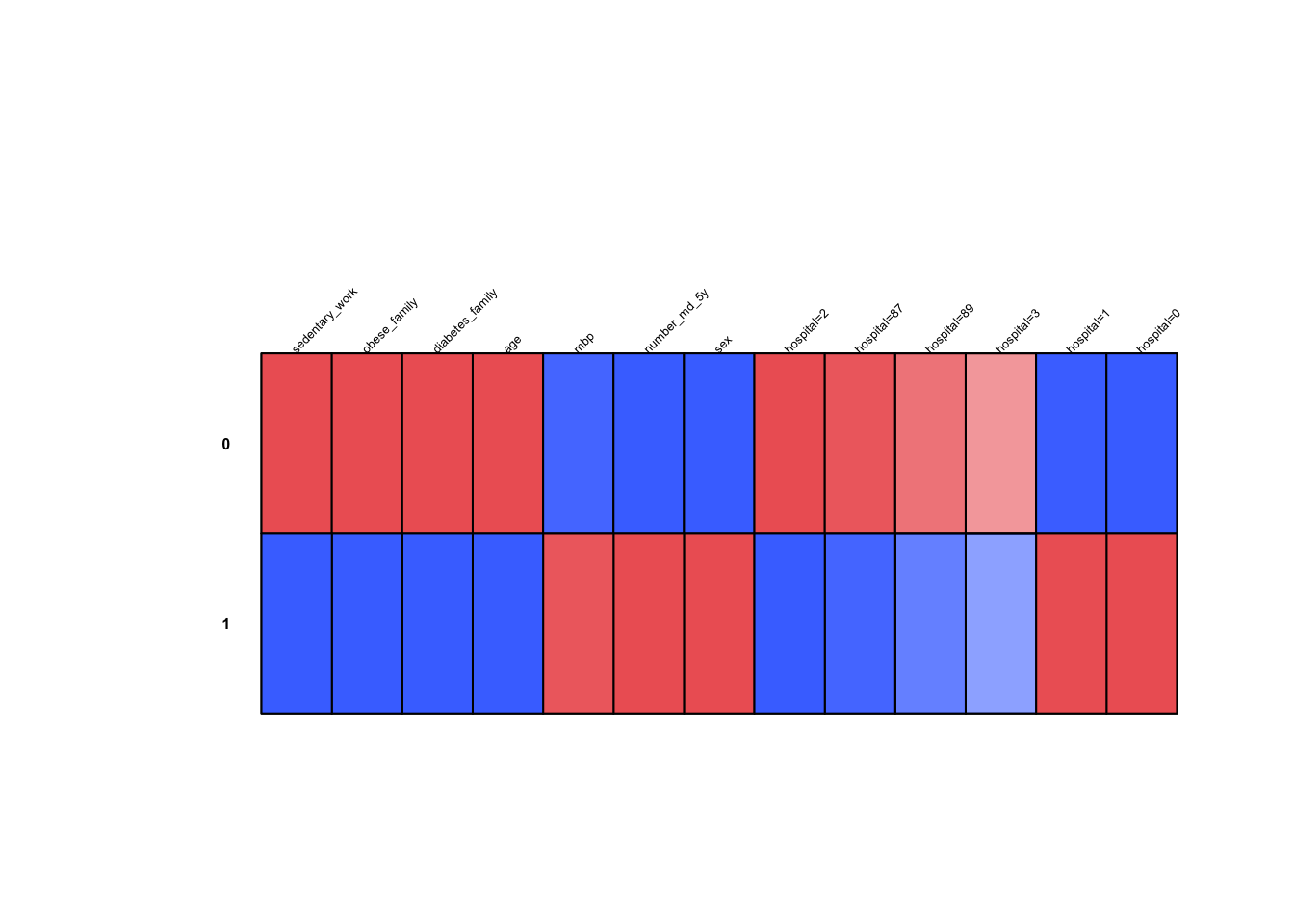control_idx <- which(dataset$W == 0) # Filter treatment / control observations, pulls outcome variable as a vector y1 <- dataset[treated_idx, "Y"] # Outcome in treatment grp y0 <- dataset[control_idx, "Y"] # Outcome in control group n1 <- sum(dataset[,"W"]) # Number of obs in treatment n0 <- sum(1 - dataset[,"W"]) # Number of obs in control # Difference in means is ATE tauhat <- mean(y1) - mean(y0) # 95% Confidence intervals se_hat <- sqrt( var(y0)/(n0) + var(y1)/(n1) ) lower_ci <- tauhat - 1.96 * se_hat upper_ci <- tauhat + 1.96 * se_hat return(c(ATE = tauhat, lower_ci = lower_ci, upper_ci = upper_ci)) } tauhat_naive <- difference_in_means(df) Look at the output: print(tauhat_naive) ## ATE lower_ci upper_ci ## -0.00597090 -0.02719679 0.01525499 # Assessing treatment bias in the data We have the information that the true ATE is -0.09, but from the naive estimation we would conclude that the treatment has no effect. This is due to the treatment bias in the data, i.e., the violation of the independence assumption recalled above. A look at the contingency table of treatment and outcome can give a first hint. 1. Using the function table, prop.table (with the option margin if needed) comment the difference between old and new distributions. Estimate in the new data P(Treatment), P(Diabetes | Treatment), P(Diabetes | Control) and comment. res.table <- table(df$W==1, df$Y==1, dnn = c("Treated","Diabetes")) prop.table(res.table) ## Diabetes ## Treated FALSE TRUE ## FALSE 0.23771252 0.50056672 ## TRUE 0.08583205 0.17588872 You can also use the option margin in prop.table. prop.table(res.table, margin=1) ## Diabetes ## Treated FALSE TRUE ## FALSE 0.3219819 0.6780181 ## TRUE 0.3279528 0.6720472 Here we retrieve the treatment effect $$\bar Y_1-\bar Y_0= 0.6720472-0.6780181$$. When we interpret a contingency table, if the percentage sum in rows, you compare the columns and vice-versa. From the above table we can also deduce the following estimates for the population characteristics: Diabetes prevalence: 67.6 % Estimated P(Treatment): 0.26 P(Diabetes | Treatment): 0.67 P(Diabetes | Control): 0.68 These quantities suggest that the treatment has a no or even a deteriorating impact on the chances of developing type 2 diabetes. We know however from the person who generated the data, that this is not the case and that the treatment has a beneficial impact. A way to assess the imbalance between the treatment and control groups is to compute and visualize the standardized mean differences in quantitative variables between the treated and the controls. It is often done in the medical community using the package cobalt: #xvars <- names(which(sapply(df_mod, is.numeric)==TRUE)) xvars <- colnames(df[, sapply(df, is.numeric)]) xvars <- xvars[!xvars %in% c("W", "Y")] tab <- CreateTableOne(vars=xvars, strat="W", data=df[, sapply(df, is.numeric)], test=FALSE) print(tab, smd=TRUE) ## Stratified by W ## 0 1 SMD ## n 7165 2540 ## number_md_5y (mean (SD)) 1.88 (0.72) 1.98 (0.75) 0.132 ## bmi (mean (SD)) 31.92 (3.58) 31.97 (3.60) 0.014 ## mbp (mean (SD)) 35.77 (10.98) 36.52 (11.28) 0.068 ## age (mean (SD)) 47.76 (13.70) 46.26 (15.04) 0.104 ## sex (mean (SD)) 0.42 (0.49) 0.76 (0.43) 0.746 ## diabetes_family (mean (SD)) 0.88 (0.33) 0.84 (0.37) 0.108 ## obese_family (mean (SD)) 0.86 (0.34) 0.75 (0.43) 0.289 ## alcohol_consumption (mean (SD)) 0.27 (0.44) 0.25 (0.43) 0.043 ## cardio_vascular_disease (mean (SD)) 0.41 (0.49) 0.40 (0.49) 0.015 ## sedentary_work (mean (SD)) 0.52 (0.50) 0.13 (0.34) 0.897 balance <- bal.tab(df[,xvars], treat = df$W, estimand="ATE", continuous="std")
love.plot(x = balance, stat = "mean.diffs", abs = TRUE, var.order = "unadjusted", threshold = 0.1, cex=0.8)This plot gives the standardized mean differences before and after weighting, i.e., the difference in means between the two groups divided by the pooled standard deviation. For a variable $$X_j$$, this corresponds to:

$SMD(X_j) = \frac{\overline{X_j^{(1)}} - \overline{X_j^{(0)}}}{\sqrt{\frac{(s^{(1)})^{2}+(s^{(0)})^{2}}{2}}}$ This SMD can be interpreted as a difference in means in standard deviation units.

Note that for binary variables, the SMD is defined via sample probabilities: if variable $$X_k$$ is binary (assume $$X_k\in\{0,1\}$$), denote by $$p_k^{(w)}$$ the probability $$Pr(X_{ik} = 1)$$ in treatment group $$w$$. Then $SMD(X_k) = \frac{\widehat{p_k}^{(1)} - \widehat{p_k}^{(0)}}{\sqrt{\frac{\widehat{p_k}^{(1)}(1-\widehat{p_k}^{(1)})+\widehat{p_k}^{(0)}(1-\widehat{p_k}^{(0)})}{2}}}$

The standardized mean differences (SMD) higher than some threshold th, for instance th=0.1, indicate that the covariate distributions in the two groups differ: the treatment is not given uniformly at random. This explains the need for some adjustment or balancing in order to perform a causal analysis of the treatment on a certain outcome.

Unsurprisingly, the two groups differ on the set of variables that we used earlier to identify likely and unlikely diabetes patients.

Similarly, you can use the function catdes of the FactoMineR package which is dedicated to the description of a categorical variable by other variables. This function is often used in clustering to quickly describe clusters.

df_Q <- df
df_Q[, "W"] <- as.factor(df_Q[, "W"])
indW <- which(colnames(df_Q)=="W")
res.cat <- catdes(df_Q, indW, proba = 0.05)
plot(res.cat, level = 0.05)For more information on catdes, you can look at the supplementary files. In addition, you can use FactoShiny: https://www.youtube.com/watch?v=JAsvTf-8cXo&list=PLnZgp6epRBbQ3UELPgndosV4dNYpT9l1-&index=4&t=0s

# Assumptions

To be able to estimate ATE from observational data, we need additional assumptions.

The first one is known as unconfoundedness, and it is formalized as a conditional independence assumption as follows.

$Y_i(1), Y_i(0) \perp W_i \ | \ X_i$

Unconfoundedness implies that the treatment is randomly assigned within each subpopulation indexed by $$X_i = x$$. Alternatively, it means that once we know all observable characteristics of individual $$i$$, then knowing about his or her treatment status gives us no extra information about their potential outcomes.

The second assumption will be called here the overlap assumption. It is formally stated like as follows.

$\forall x \in \text{supp}(X), \qquad 0 < P(W = 1 \ | \ X = x) < 1$

Effectively, this assumption guarantees that no subpopulation indexed by $$X=x$$ is entirely located in only one of control or treatment groups. It is necessary to ensure that we are able to compare individuals in control and treatment for every subpopulation.

Finally, the conditional probability of treatment given controls $$P(W=1|X=x)$$ is called the propensity score. In the next sections, it will play a central role in estimation and inference of causal effects. The propensity score can be estimated by any methods you prefer, and it’s always a good idea to check that the estimated propensity score satisfies the overlap assumption. For example, let’s estimate it using a logistic regression.

1. Computing the propensity score by logistic regression of W on X and comment on the overlap assumption using an appropriate representation.
# Computing the propensity score by logistic regression of W on X.
p_logistic.fit <- glm(W ~ ., data = dplyr::select(df, -c("Y")), family = binomial(link="logit"))
p_logistic <- as.vector(predict(p_logistic.fit, type = "response"))
# Not to forget, type = response is to get the probabilities $p_i = exp(X\beta)/1+exp(X\beta)$ otherwise, R outputs the logit(p_i)

hist(p_logistic, main = "Histogram: Logistic Regression Propensity Scores"
, xlab = "Propensity Score", col = "cornflowerblue", freq = FALSE)